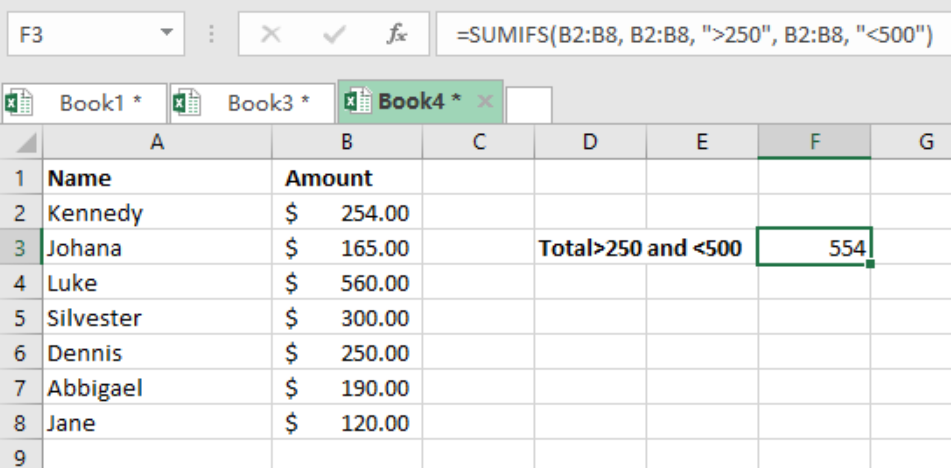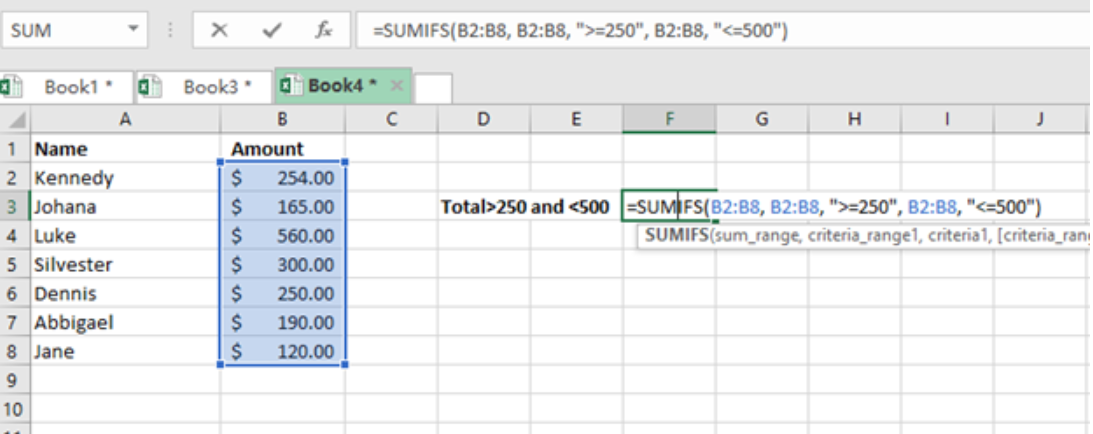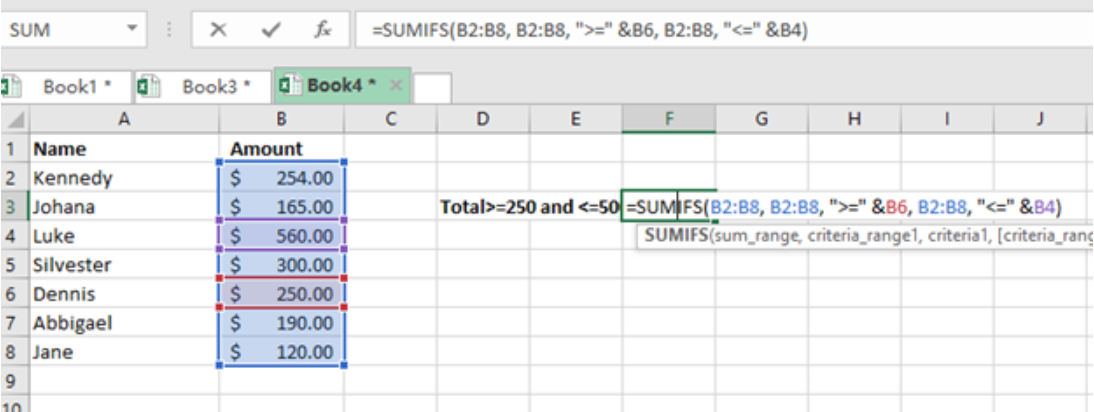Get instant live expert help with Excel or Google Sheets“My Excelchat expert helped me in less than 20 minutes, saving me what would have been 5 hours of work!”

#### Post your problem and you'll get expert help in seconds

Your message must be at least 40 characters
Our professional experts are available now. Your privacy is guaranteed.

# Sum if between

Excel conditions sum and if can be combined to form sum if between conditions. This combination is necessary for adding cells or inputs which satisfy two or more criteria between given cell ranges in a sheet. This article provides a guide on how to use the sum if between conditions in excel.Figure 1. Final result

## Syntax of the formula

`=SUMIFS(sum-range, criteria-range, “>criteria1”, criteria-range, “<criteria2”)`

This formula works with more than one criteria. In the case above, we have the first criteria as the amount being >250 while the second one being <500. Note that the amount in the syntax refers to the named range with the amount, which is B2:B8.

## Explanation

SUMIFS function works with many logical operators in excel. These include “=”, “>”, “<”, “>=” and many others.

In our example above, we want to find the sum of the amount that is above \$250 but less than \$500. These are values found in column B. The sum_range will thus be B2:B8.

With the criteria in place, the Excel built-in function SUMIFS will sums up all the values that are greater than \$250 but less than \$500.

Note that our thresholds, “\$250” and “\$500” are excluded from the result. If you want them included, then you should replace “<” and “>” with “<=” and “>=” respectively.

## ExampleFigure 2. Example

## Using cell reference

We can also use cell reference in order to easily expose the threshold and easily change the amounts. This can be done using the formula below:

`=SUMIFS(amount, amount, “>=” &A1, amount, “<”&B1)`

In this case, A1 refers to lower threshold while B1 to upper threshold.

### ExampleFigure 3. Cell reference

## Instant Connection to an Expert through our Excelchat Service

Most of the time, the problem you will need to solve will be more complex than a simple application of a formula or function. If you want to save hours of research and frustration, try our live Excelchat service! Our Excel Experts are available 24/7 to answer any Excel question you may have. We guarantee a connection within 30 seconds and a customized solution within 20 minutes.

### Did this post not answer your question? Get a solution from connecting with the expert.Another blog reader asked this question today on Excelchat:## Subscribe to Excelchat.coAnother blog reader asked this question today on Excelchat: